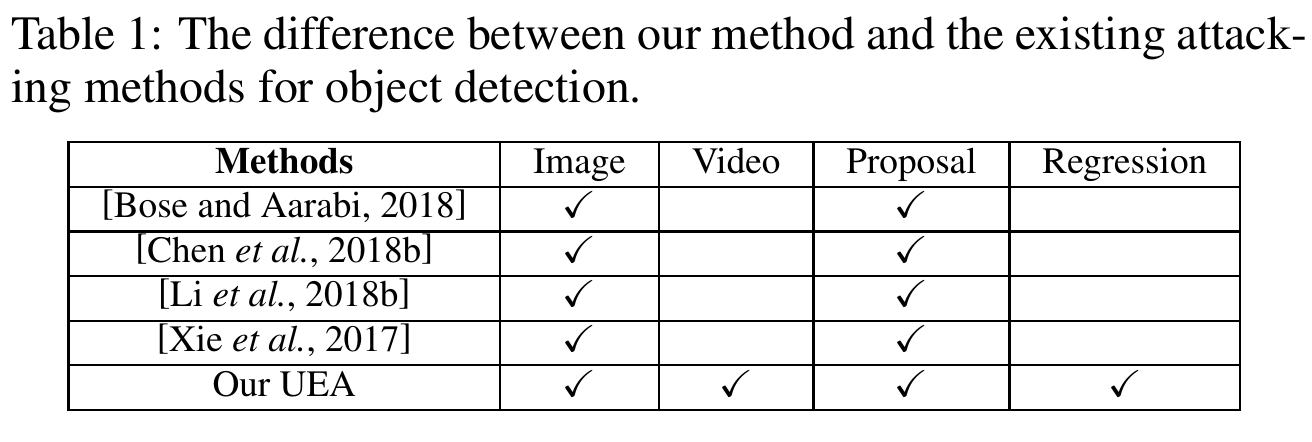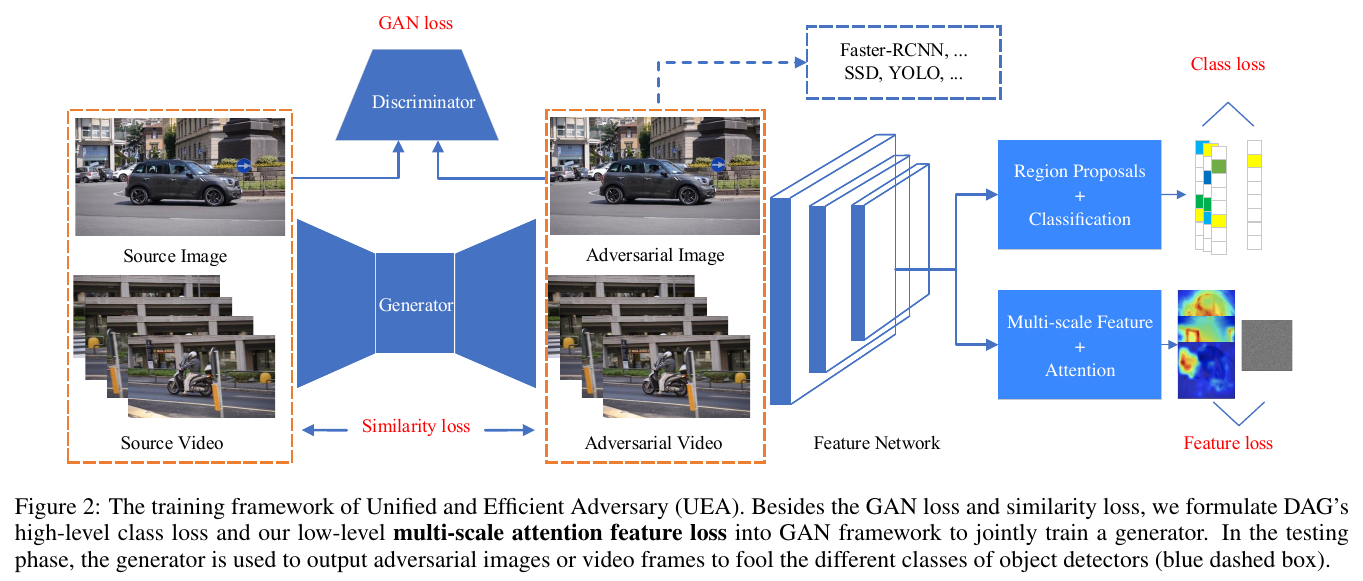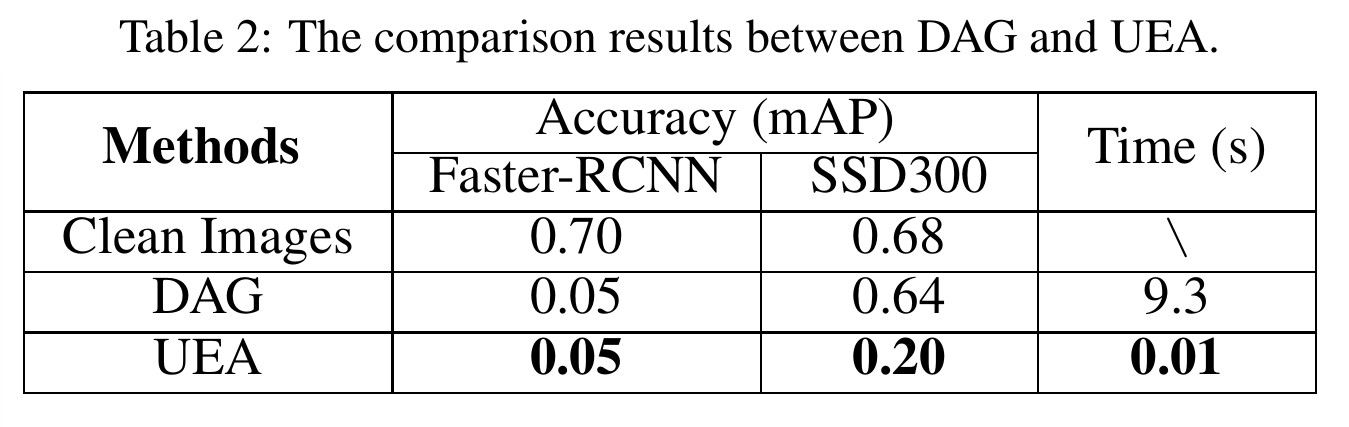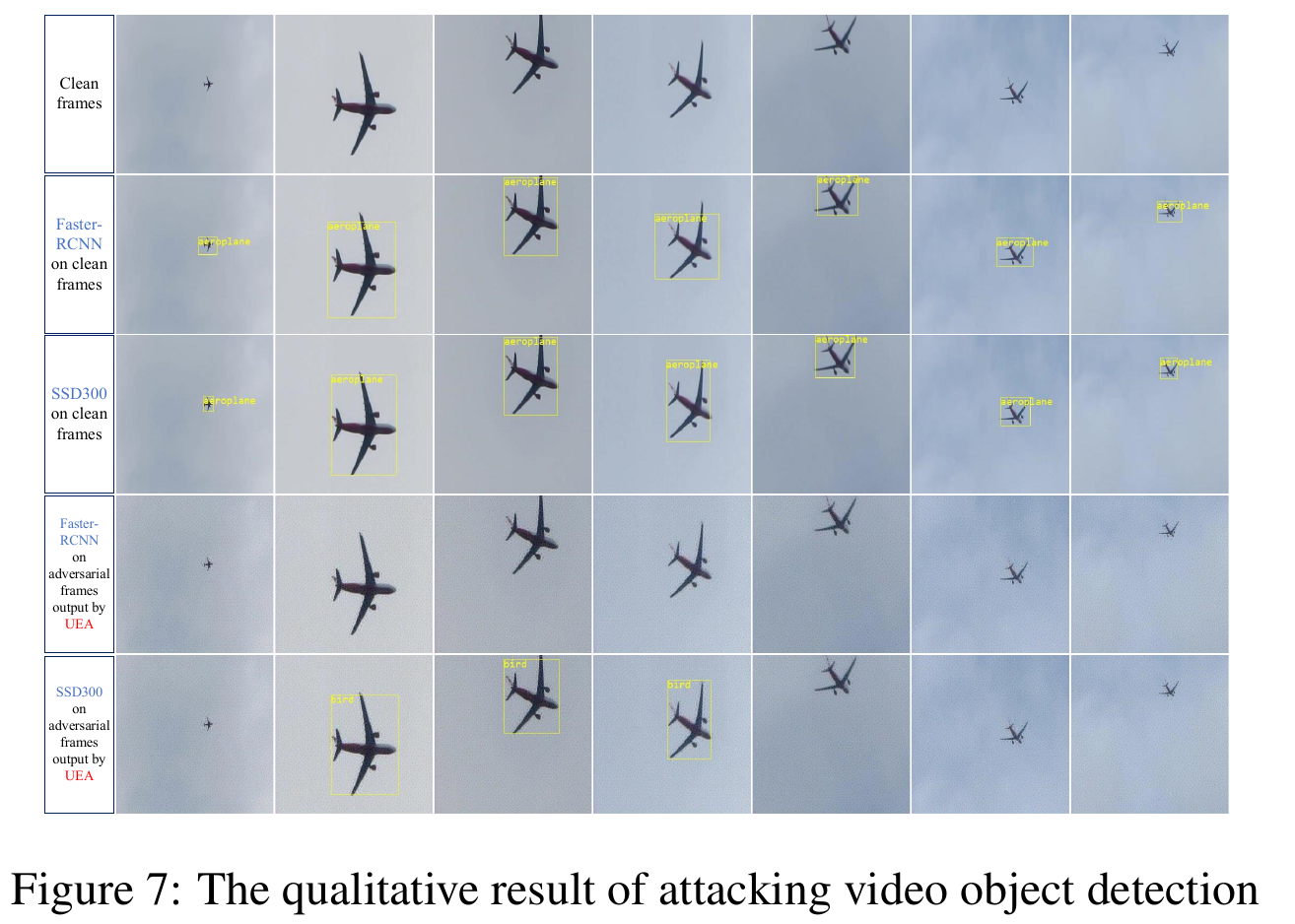# 论文阅读-可迁移对抗样本攻击

Hello 大家好，本次带来的是针对目标检测的可迁移对抗样本攻击。

### 1. 前情提要不管你哪种方法，你总要有 feature map 的吧？那我把 feature map 也给攻击了，岂不就是一个大一统的攻击方法了？

### 2. UEA（Unified and Efficient Adversary）$L_{cGAN}(G,D) = E_I[logD(I)]+E_I[log(1-D(G(I)))]$

$L_{L_2}(G) = E_I[||I-G(I)||_2]$

$L_{DAG}(G) = E_I[\sum_{n=1}^N[f_{l_n}(X,t_n) - f_{\hat{l}_n}(X,t_n)]$

ground-truth label， $\hat{l}_n$ 是从其他错误 labels 里随机取样的错误 label，$f_{l_n}(X,t_n)\in\mathbb{R}^{C}$

$L_{Fea}(G) = E_[\sum_{m=1}^M||A_m*(X_m-R_m)||_2]$

$L= L_{cGAN} + \alpha L_{L_2} + \beta L_{DAG} + \epsilon L_{Fea}$

### 3. 大功告成regards.

#### oukohou.

©️2019 CSDN 皮肤主题: 大白 设计师: CSDN官方博客